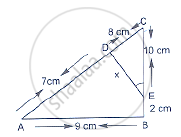Share

Books Shortlist
Your shortlist is empty

# Solution for In Below Figure, ∠A = ∠Ced, Prove that δCab ~ δCed. Also, Find the Value of X. - CBSE Class 10 - Mathematics

ConceptCriteria for Similarity of Triangles

#### Question

In below figure, ∠A = ∠CED, Prove that ΔCAB ~ ΔCED. Also, find the value of x.#### Solution

We have, ∠A = ∠CED

In ΔCAB and ΔCED

∠C = ∠C                               [Common]

∠A = ∠CED                             [Given]

Then, ΔCAB ~ ΔCED                  [By AA similarity]

therefore"CA"/"CE"="AB"/"ED"                 [Corresponding parts of similar Δ are proportional]

rArr15/10=9/x

rArrx=(10xx9)/15=90/15=6  cm

Is there an error in this question or solution?

#### Video TutorialsVIEW ALL 

Solution for question: In Below Figure, ∠A = ∠Ced, Prove that δCab ~ δCed. Also, Find the Value of X. concept: Criteria for Similarity of Triangles. For the course CBSE
S